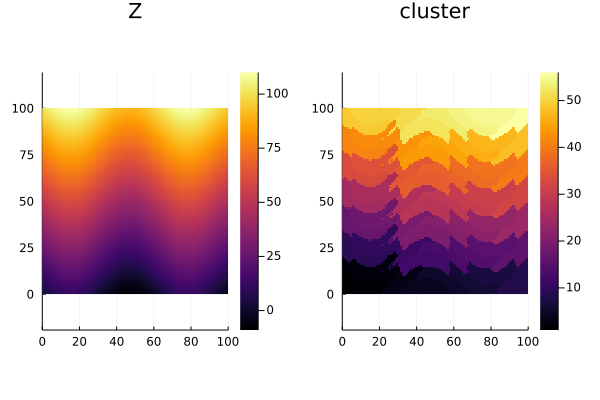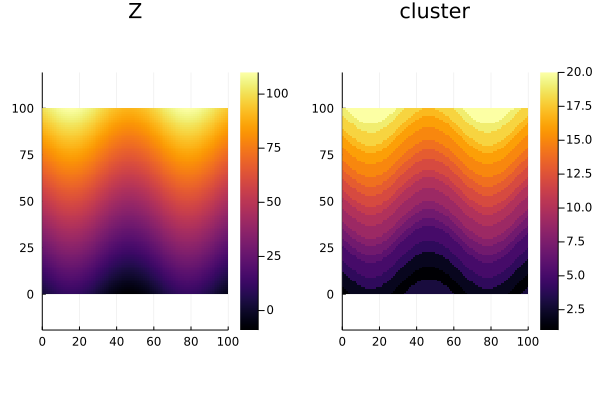# Clustering

Geostatistical clustering (a.k.a. domaining) is the process of partitioning geospatial data in terms of both features and geospatial coordinates. Below is the current list of clustering methods available:

## SLIC

GeoClustering.SLICType
SLIC(k, m; tol=1e-4, maxiter=10)

A method for clustering geospatial data into approximately k clusters using Simple Linear Iterative Clustering (SLIC). The method produces clusters of samples that are spatially connected based on a distance dₛ and that, at the same time, are similar in terms of vars with distance dᵥ. The tradeoff is controlled with a hyperparameter parameter m in an additive model dₜ = √(dᵥ² + m²(dₛ/s)²).

Parameters

• k - Approximate number of clusters
• m - Hyperparameter of SLIC model
• tol - Tolerance of k-means algorithm (default to 1e-4)
• maxiter - Maximum number of iterations (default to 10)
• weights - Dictionary with weights for each attribute (default to nothing)

References

Ω = georef((Z=[10sin(i/10) + j for i in 1:100, j in 1:100],))

C = cluster(Ω, SLIC(50, 0.01))

plot(plot(Ω), plot(C))## GHC

GeoClustering.GHCType
GHC(k, λ; kern=:epanechnikov, link=:ward)

A method for partitioning spatial data into k clusters according to a range λ using Geostatistical Hierarchical Clustering (GHC). The larger the range the more connected are nearby samples.

Parameters

• k - Approximate number of clusters
• λ - Approximate range of kernel function
• kern - Kernel function (:uniform, :triangular or :epanechnikov)
• link - Linkage function (:single, :average, :complete, :ward or :ward_presquared)

References

Notes

• The range parameter controls the sparsity pattern of the pairwise distances, which can greatly affect the computational performance of the GHC algorithm. We recommend choosing a range that is small enough to connect nearby samples. For example, clustering data over a 100x100 Cartesian grid with unit spacing is possible with λ=1.0 or λ=2.0 but the problem starts to become computationally unfeasible around λ=10.0 due to the density of points.
C = cluster(Ω, GHC(20, 1.0))

plot(plot(Ω), plot(C))